# Wavelet Transforms¶

New in version 0.9.1: Wavelet functions were only added in version 0.9.1

We are going to use wavelets to transform an image so that most of its values are 0 (and otherwise small), but most of the signal is preserved.

The code for this tutorial is avalailable from the source distribution as `mahotas/demos/wavelet_compression.py`.

```import numpy as np
import mahotas
import mahotas.demos

from mahotas.thresholding import soft_threshold
from matplotlib import pyplot as plt
from os import path
f = f[:256,:256]
plt.gray()
# Show the data:
print("Fraction of zeros in original image: {0}".format(np.mean(f==0)))
plt.imshow(f)
plt.show()
```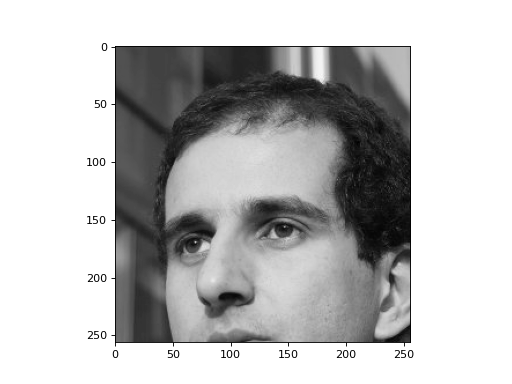There are no zeros in the original image. We now try a baseline compression method: save every other pixel and only high-order bits.

```direct = f[::2,::2].copy()
direct /= 8
direct = direct.astype(np.uint8)
print("Fraction of zeros in original image (after division by 8): {0}".format(np.mean(direct==0)))
plt.imshow(direct)
plt.show()
```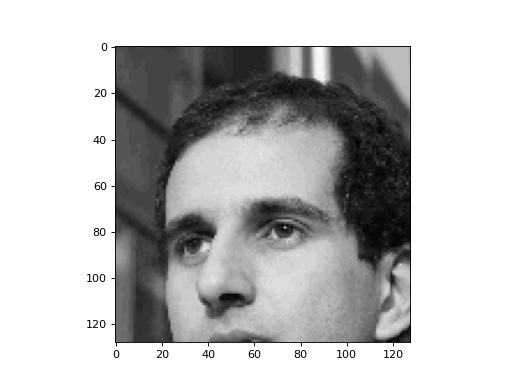There are only a few zeros, though. We have, however, thrown away 75% of the values. Can we get a better image, using the same number of values, though?

We will transform the image using a Daubechies wavelet (D8) and then discard the high-order bits.

```# Transform using D8 Wavelet to obtain transformed image t:
t = mahotas.daubechies(f,'D8')

t /= 8
t = t.astype(np.int8)
print("Fraction of zeros in transform (after division by 8): {0}".format(np.mean(t==0)))
plt.imshow(t)
plt.show()
```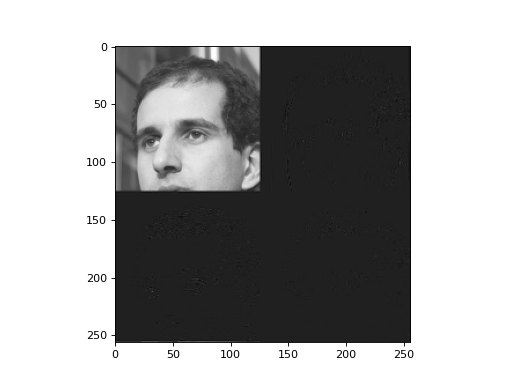This has 60% zeros! What does the reconstructed image look like?

```# Let us look at what this looks like
r = mahotas.idaubechies(t, 'D8')
plt.imshow(r)
plt.show()
```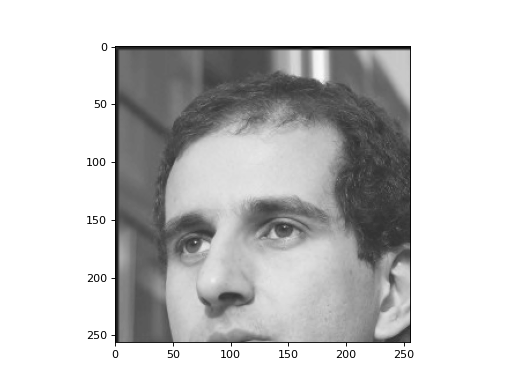This is a pretty good reduction without much quality loss. We can go further and discard small values in the transformed space. Also, let’s make the remaining values even smaller in magnitude.

Now, this will be 77% of zeros, with the remaining being small values. This image would compress very well as a lossless image and we could reconstruct the full image after transmission. The quality is certainly higher than just keeping every fourth pixel and low-order bits.

```tt = soft_threshold(t, 12)
print("Fraction of zeros in transform (after division by 8 & soft thresholding): {0}".format(np.mean(tt==0)))
# Let us look again at what we have:
rt = mahotas.idaubechies(tt, 'D8')
plt.imshow(rt)
```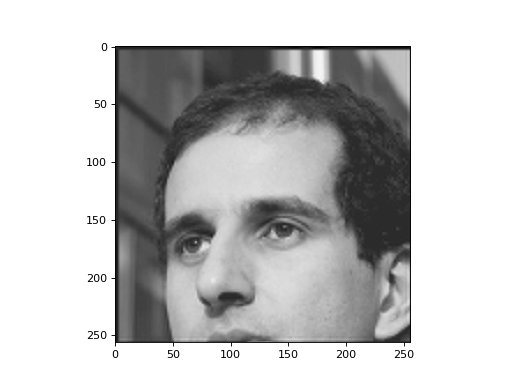In this example, we can see some artifacts at the border. We can use `wavelet_center` and `wavelet_decenter` to handle borders to correctly:

```fc = mahotas.wavelet_center(f)
t = mahotas.daubechies(fc, 'D8')
r = mahotas.idaubechies(fc, 'D8')
rd = mahotas.wavelet_decenter(r, fc.shape)
```

Now, `rd` is equal (except for rounding) to `fc` without any border effects.

## API Documentation¶

A package for computer vision in Python.

### Main Features¶

features
Compute global and local features (several submodules, include SURF and Haralick features)
convolve
Convolution and wavelets
morph
Morphological features. Most are available at the mahotas level, include erode(), dilate()...
watershed
Seeded watershed implementation

Citation:

Coelho, Luis Pedro, 2013. Mahotas: Open source software for scriptable computer vision. Journal of Open Research Software, 1:e3, DOI: http://dx.doi.org/10.5334/jors.ac
`mahotas.``haar`(f, preserve_energy=True, inline=False)

Haar transform

Parameters: f : 2-D ndarray Input image preserve_energy : bool, optional Whether to normalise the result so that energy is preserved (the default). inline : bool, optional Whether to write the results to the input image. By default, a new image is returned. Integer images are always converted to floating point and copied.

`ihaar`
function Reverse Haar transform
`mahotas.``ihaar`(f, preserve_energy=True, inline=False)

Reverse Haar transform

`ihaar(haar(f))` is more or less equal to `f` (equal, except for possible rounding issues).

Parameters: f : 2-D ndarray Input image. If it is an integer image, it is converted to floating point (double). preserve_energy : bool, optional Whether to normalise the result so that energy is preserved (the default). inline : bool, optional Whether to write the results to the input image. By default, a new image is returned. Integer images are always converted to floating point and copied. f : ndarray

`haar`
function Forward Haar transform
`mahotas.``daubechies`(f, code, inline=False)

Daubechies wavelet transform

This function works best if the image sizes are powers of 2!

Parameters: f : ndarray 2-D image code : str One of ‘D2’, ‘D4’, ... ‘D20’ inline : bool, optional Whether to write the results to the input image. By default, a new image is returned. Integer images are always converted to floating point and copied.

`haar`
`mahotas.``idaubechies`(f, code, inline=False)
`haar`